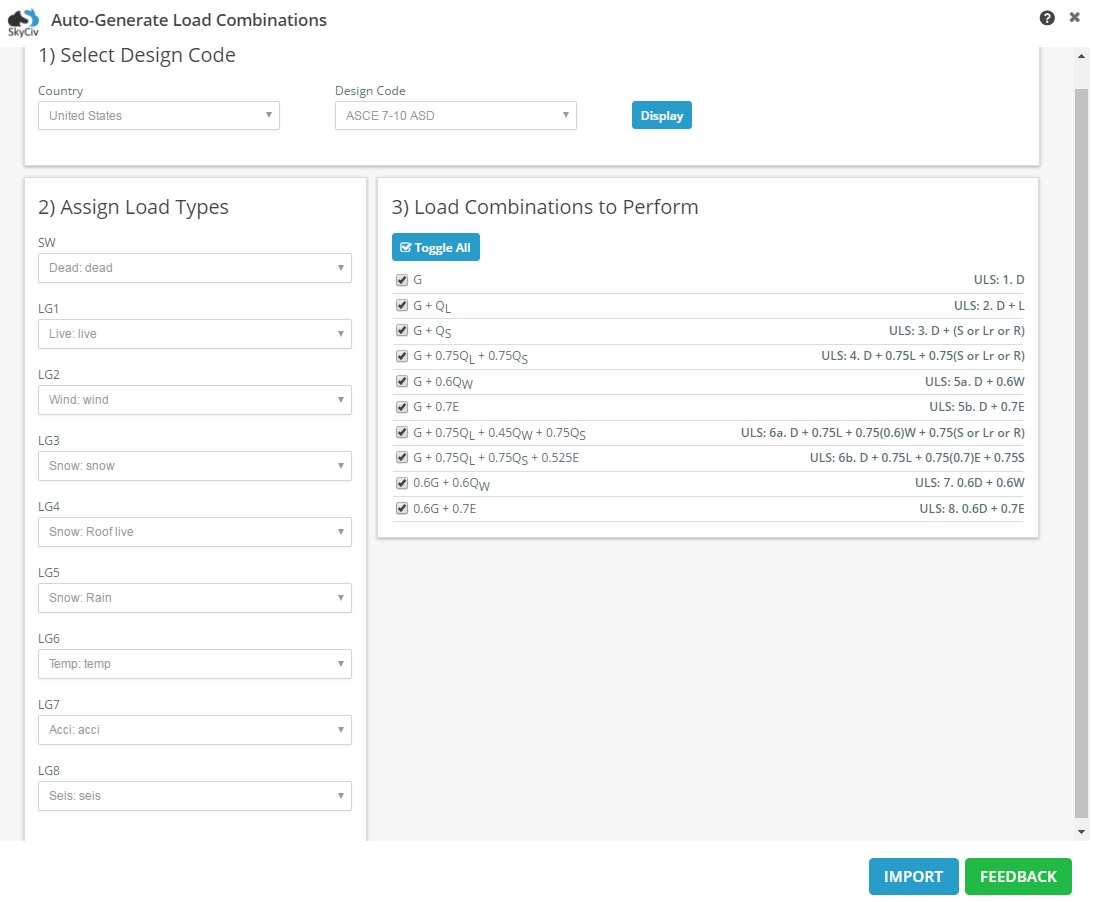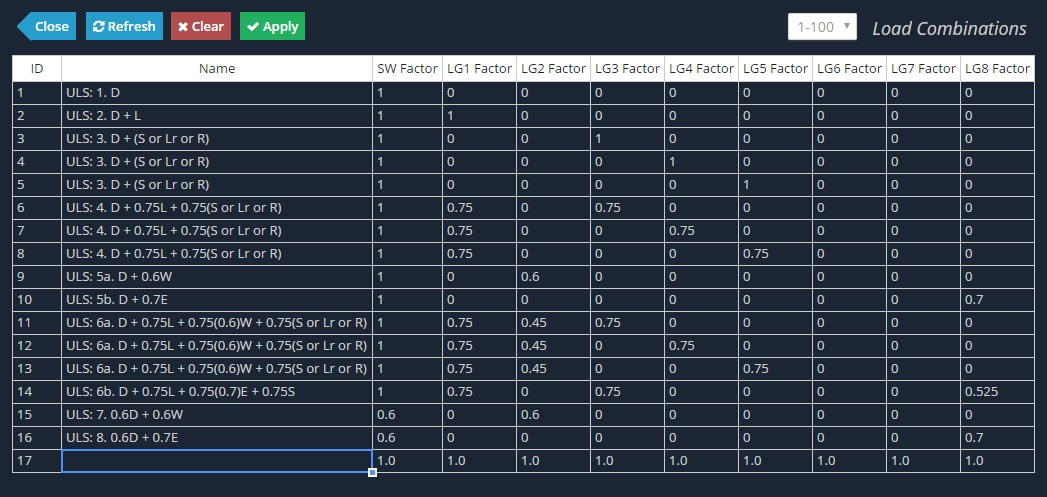Documentation [Deprecated]
SkyCiv Documentation

Getting Started

Section Builder
Operations
Built-Up and Composite Sections
Custom Shapes

Member Design
General
Member Design Modules
Code Verification

RC Design
General
Code Verification

# Load Combinations: ASCE 7-10 ASD

In the United States, ASCE 7-10 is an key part of the building code. It focuses on the requirements for general structural design, as well as providing a means for determining loads (dead, live, soil, flood, snow, rain, ice, earthquake, wind) and their combinations.

Allowable Strength Design (ASD) involves eight basic load combination equations.

This article will focus on how SkyCiv’s auto generated load combinations feature meets the load combination equations as specified in ASCE 7-10 ASD.

## 2.4.1 Basic Combinations

Design Code Equation Design Code Comment SkyCiv Equation SkyCiv Comment
D Eq. 2.4.1.1 G -
D + L Eq. 2.4.1.2 G + QL -
D + (Lr or S or R) Eq. 2.4.1.3 G + QS The load type QS "Snow" is sub-categorized into "snow", "roof live", and "rain". Selecting this load combination will automatically create 3 load combination equations due to the use of "or" in the equation specification.
D + 0.75L + 0.75(Lr or S or R) Eq. 2.4.1.4 G + 0.75QL + 0.75QS The load type QS "Snow" is sub-categorized into "snow", "roof live", and "rain". Selecting this load combination will automatically create 3 load combination equations due to the use of "or" in the equation specification.
D + (0.6W or 0.7E) Eq. 2.4.1.5 G + 0.6QW
G + 0.7E
Due to the presence of an "or" in the load combination, two load combination eqations are required to represent them. Since "wind" and "seismic" are not linked by a load type, two separate equations are given by SkyCiv, instead of auto-generating equations in the case of the QS snow load type.
D + 0.75L + 0.75(0.6W) + 0.75(Lr or S or R) Eq. 2.4.1.6a G + 0.75QL + 0.45QW + 0.75QS The load type QS "Snow" is sub-categorized into "snow", "roof live", and "rain". Selecting this load combination will automatically create 3 load combination equations due to the use of "or" in the equation specification.
D + 0.75L + 0.75(0.7E) + 0.75S Eq. 2.4.1.6b G + 0.75QL + 0.75QS + 0.525E Only one load equation is required to represent this load combination. Despite the presence of QS "Snow" load type, only one equation is generated by SkyCiv for the "snow" sub-type.
0.6D + 0.6W Eq. 2.4.1.7 0.6G + 0.6QW -
0.6D + 0.7E Eq. 2.4.1.8 0.6G + 0.7E -

## Example: All Load Types Assigned

Whilst not practical, 9 load groups have been created and assigned to all 9 load types as seen below. This is done to check the equations that are formed from the load combinationsThe results from the datasheet are shown below.